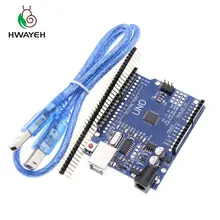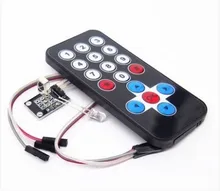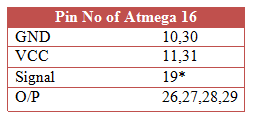IR Based Home Automation Using Arduino | PC Week

# IR Based Home Automation Using ArduinoHome Automation is always a bit costlier and not affordable for ordinary people because of its cost and availability of technicians. There are various types of Automation technique which are opted with convenience and budget.

But today we are talking about an Automation technique where we can use our old TV remote to Control our Home Appliances.

In this article, We will learn how to make a cheap IR Based Home Automation Using Arduino and other components. So let start our tutorial with a quick introduction about the circuitry and program.

## IR Based Home Automation Using Arduino

Automation using Arduino is quite easier than opting for any other Platform because it is beginner friendly and cheap. We can use any of the Arduino boards, even bare Atmel Microcontroller can be used to create a compact Automation kit.

For working with bare MCU and Arduino first of all you should need to bootload the Arduino with preferred Bootloader. So there are many tutorials on Automation using Arduino Uno, Nano and Micro etc

We can work with an Unofficial MCU like Atmega 16 which is enough for our IR automation project. So you will need to bootload the Atmega 16 with Bootloader first.

For that just visit the following link hereArduino Kit ( Below 1\$) Check Here

### Materials RequiredYou can use any of the MCU packages but it is easy with the DIP package and if you don't want use any external crystal Oscillator you can Avoid the 16 Mhz Oscillator with the inbuilt Internal 8 Mhz which is enough for this project.IR Arduino Kit ( Below 1\$) Check Here

### Circuit DiagramYou can also use a Bare relay with an NPN Transistor along with the MCU.

1. Carefully connect all the components just like the above circuit diagram
2. Connect VCC and GND to both MCU and IR Reciever and other Pins correctly
3. Double check circuits for any short circuit or loss connectionsSignal wire may vary according to MCU. For details about supported Pin number of your MCU. Goto this link

#### Programming

Before Programming, you have to decode each button value of your remote. If you don't know to decode the DEC value of your Remote Controller Please follow the below procedure. This should be done after Installing the below Library.

Upload this code and press the Key which you want to decode.
`-----------------------------------------------------------`
`#include <IRremote.h>`
`int IRPIN = 8;`
`IRrecv irrecv(IRPIN);`
`decode_results result;`
`void setup()`
`{`
`  Serial.begin(9600);`
`  Serial.println("Enabling IRin");`
`  irrecv.enableIRIn();`
`  Serial.println("Enabled IRin");`
`}`
`void loop() `
`{`
` if (irrecv.decode(&result)) `
`  {`
`    Serial.println(result.value, DEC);`
`    irrecv.resume(); `
`  }`
`  delay(500);`
`}`
`-----------------------------------------------------------`

After that Upload this code replacing the green coloured code with the Decoded button value of your remote control

`-----------------------------------------------------------`
`// IR Home Automation Using Arduino Version 0.1`
`#include <IRremote.h>`
`int RECV_PIN = 13; // the pin where you connect the output pin of TSOP4838`
`int led1 = 23; // Pin were devices can be connected`
`int led2 = 22; // Pin were devices can be connected`
`int led3 = 21; // Pin were devices can be connected`
`int led4 = 20; // Pin were devices can be connected`
`int led5 = 19; // Pin were devices can be connected`
`int itsONled[] = {0,0,0,0,0,0}; // Setting the initial condition of LED Off`
`#define code1  33444015 // code received from button A`
`#define code2  33478695 // code received from button B`
`#define code3  33486855 // code received from button C`
`#define code4  33435855 // code received from button D`
`#define code5  33468495 // code received from button E`
`IRrecv irrecv(RECV_PIN);`
`decode_results results;`
`void setup()`
`{`
`  Serial.begin(9600);   // Can delete this line if serial communication is not needed`
`  irrecv.enableIRIn();  // Start the receiver`
`  pinMode(led1, OUTPUT);`
`  pinMode(led2, OUTPUT);`
`  pinMode(led3, OUTPUT);`
`  pinMode(led4, OUTPUT);`
`  pinMode(led5, OUTPUT);`
`}`
`    void loop() {`
`  if (irrecv.decode(&results)) {`
`  unsigned int value = results.value;`
`  switch(value) {`
`  case code1:`
`  if(itsONled == 1) {        // if first led is on then`
`  digitalWrite(led1, LOW);   // turn it off when button is pressed`
`  itsONled = 0;           // and set its state as off`
`  } else {                      // else if first led is off`
`  digitalWrite(led1, HIGH); // turn it on when the button is pressed`
`  itsONled = 1;          // and set its state as on`
`  }`
`  break; `
`  case code2:`
`  if(itsONled == 1) {`
`  digitalWrite(led2, LOW);`
`  itsONled = 0;`
`  } else {`
`  digitalWrite(led2, HIGH);`
`  itsONled = 1;`
`  }`
`  break;`
`  case code3:`
`  if(itsONled == 1) {`
`  digitalWrite(led3, LOW);`
`  itsONled = 0;`
`  } else {`
`  digitalWrite(led3, HIGH);`
`  itsONled = 1;`
`  }`
`  break;`
`  case code4:`
`  if(itsONled == 1) {`
`  digitalWrite(led4, LOW);`
`  itsONled = 0;`
`  } else {`
`  digitalWrite(led4, HIGH);`
`  itsONled = 1;`
`  }`
`  break;`
`  case code5:`
`  if(itsONled == 1) {`
`  digitalWrite(led5, LOW);`
`  itsONled = 0;`
`  } else {`
`  digitalWrite(led5, HIGH);`
`  itsONled = 1;`
`  }`
`  break;`
`  }`
`  Serial.println(value); // Can delete this line if serial communication is not needed`
`  irrecv.resume(); // Receive the next value`
`  }`
`}`
`-----------------------------------------------------------`

If You get any Error while uploading or Compiling the Project then Double check the ISP connection and Make sure that you Installed the library

Development of these project is carried out here, You can too Contribute to this Project!

## Conclusion

We hope that you liked this article on  IR Based Home Automation Using Arduino. If you have any doubt and problem please comment below. We are happy to help you!